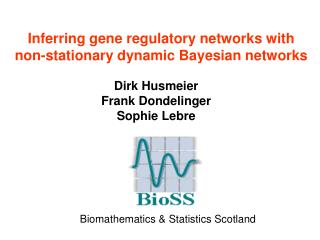DownloadDownload PresentationInferring gene regulatory networks with non-stationary dynamic Bayesian networks

# Inferring gene regulatory networks with non-stationary dynamic Bayesian networks

Download Presentation## Inferring gene regulatory networks with non-stationary dynamic Bayesian networks

- - - - - - - - - - - - - - - - - - - - - - - - - - - E N D - - - - - - - - - - - - - - - - - - - - - - - - - - -
##### Presentation Transcript

1. Dirk Husmeier Frank Dondelinger Sophie Lebre Inferring gene regulatory networks with non-stationary dynamic Bayesian networks Biomathematics & Statistics Scotland

2. Overview • Introduction • Non-homogeneous dynamic Bayesian network for non-stationary processes • Flexible network structure • Open problems

3. Can we learn signalling pathways from postgenomic data? From Sachs et al Science 2005

4. Network reconstruction from postgenomic data

5. Marriage between graph theory and probability theory Friedman et al. (2000), J. Comp. Biol. 7, 601-620

6. Bayes net ODE model

7. Graph theory • Directed acyclic graph (DAG) representing conditional independence relations. • Probability theory • It is possible to score a network in light of the data: P(D|M), D:data, M: network structure. • We can infer how well a particular network explains the observed data. NODES A B C EDGES D E F

8. BGe (Linear model) [A]= w1[P1]+ w2[P2] + w3[P3] + w4[P4] + noise P1 w1 P2 A w2 w3 P3 w4 P4

9. BDe (Nonlinear discretized model) P P1 Activator P2 Activation Repressor Allow for noise: probabilities P P1 Activator P2 Inhibition Conditional multinomial distribution Repressor

10. Model Parameters q Integral analytically tractable!

11. BDe: UAI 1994 BGe: UAI 1995

12. Dynamic Bayesian network

13. Example: 2 genes 16 different network structures Best network: maximum score

14. Identify the best network structure Ideal scenario: Large data sets, low noise

15. Uncertainty about the best network structure Limited number of experimental replications, high noise

16. Sample of high-scoring networks

17. Sample of high-scoring networks Feature extraction, e.g. marginal posterior probabilities of the edges

18. Sample of high-scoring networks Feature extraction, e.g. marginal posterior probabilities of the edges Uncertainty about edges High-confident edge High-confident non-edge

19. Can we generalize this scheme to more than 2 genes? In principle yes. However …

20. Number of structures Number of nodes

21. Sampling from the posterior distribution Find the high-scoring structures Taken from the MSc thesis by Ben Calderhead Configuration space of network structures

22. Madigan & York (1995), Guidici & Castello (2003)

23. Local change MCMC If accept If accept with probability Taken from the MSc thesis by Ben Calderhead Configuration space of network structures

24. Overview • Introduction • Non-homogeneous dynamic Bayesian networks for non-stationary processes • Flexible network structure • Open problems

25. Dynamic Bayesian network

26. Example: 4 genes, 10 time points

27. Standard dynamic Bayesian network: homogeneous model

28. Limitations of the homogeneity assumption

29. Learning with MCMC q Allocation vector h k Number of components (here: 3)

30. Non-homogeneous model  Non-linear model

31. BGe: Linear model [A]= w1[P1]+ w2[P2] + w3[P3] + w4[P4] + noise P1 w1 P2 A w2 w3 P3 w4 P4

32. BDe: Nonlinear discretized model P P1 Activator P2 Activation Repressor Allow for noise: probabilities P P1 Activator P2 Inhibition Conditional multinomial distribution Repressor

33. Linear Gaussian model Restriction to linear processes Original data  no information loss Multinomial model Nonlinear model Discretization  information loss Pros and cons of the two models

34. Can we get an approximate nonlinear model without data discretization? y x

35. Can we get an approximate nonlinear model without data discretization? Idea: piecewise linear model y x

36. NIPS 2009

37. Overview • Introduction • Non-homogeneous dynamic Bayesian network for non-stationary processes • Flexible network structure • Open problems

38. Non-stationarity in the regulatory process

39. Non-stationarity in the network structure

40. ICML 2010

41. Flexible network structure with regularization

42. Flexible network structure with regularization

43. Flexible network structure with regularization

44. Morphogenesis in Drosophila melanogaster • Gene expression measurements over 66 time steps of 4028 genes (Arbeitman et al., Science, 2002). • Selection of 11 genes involved in muscle development. Zhao et al. (2006), Bioinformatics22

45. Transition probabilities: flexible structure with regularization Morphogenetic transitions: Embryo  larva larva pupa pupa  adult